# Wheel Rate and Chassis Roll StiffnessWheel Rate

Wheel rate is effectively the spring rate when measured at the wheel as opposed to at the spring. It is important to understand the wheel rate of a vehicle for calculating the spring rates and understanding the car dynamically.

Coil Spring Wheel Rate

In order to calculate wheel rate we need to know a few things:

• Installation Ratio
• Spring Rate
• Damper Angle from Vertical (shown in the image below)Some dampers are mounted vertically from the front view but are at an angle from the side view like in the image below. This angle from vertical should be used instead if that is the case.These 3 factors can then be used in an equation below to calculate the wheel rate of a car.Where:

• Kwheel = Wheel Rate
• K = Spring Rate
• IR = Installation Ratio
• Φ = Damper Angle from Vertical.

So using the below example values we can calculate the wheel rate for our car:

• K = 61484 N/m
• IR = 0.6
• Φ = 10 degrees

Therefore:So:This is the value for one of your axles (front or rear) now repeat the above process to calculate the wheel rate for your other axle. For example, above we have used our front spring rates and front coilover installation ratios. This now needs to be re-done with our rear spring rates and rear coilover installation ratios to calculate our rear wheel rate.

This is our value for wheel rate during bump. In order to calculate our wheel rate in roll we must now calculate the wheel rate for our anti-roll bar.

Anti-Roll Bar Wheel Rate

With the anti-roll bar spring rates calculated in the first stage we have a value in N/m. Therefore we can put this into a similar equation as for the coil springs to produce the wheel rate for the anti-roll bar. The equation is:Where:

• Kwheel = Wheel Rate
• K = Anti-Roll Bar Spring Rate
• IR = Anti-Roll Bar Installation Ratio
• Φ = Drop link Angle from Vertical (shown in the image below)So using the below example values we can calculate the wheel rate for our car:

• K = 36448 N/m
• IR = 0.81
• Φ = 0 degrees

Therefore:So:This is the value for one of your axles (front or rear) now repeat the above process to calculate the wheel rate for your other axle. For example, above we have used our front anti-roll bar spring rate and front anti-roll bar installation ratio. This now needs to be re-done with our rear anti-roll bar spring rate and rear anti-roll bar installation ratio to calculate our rear wheel rate.

Chassis Roll Stiffness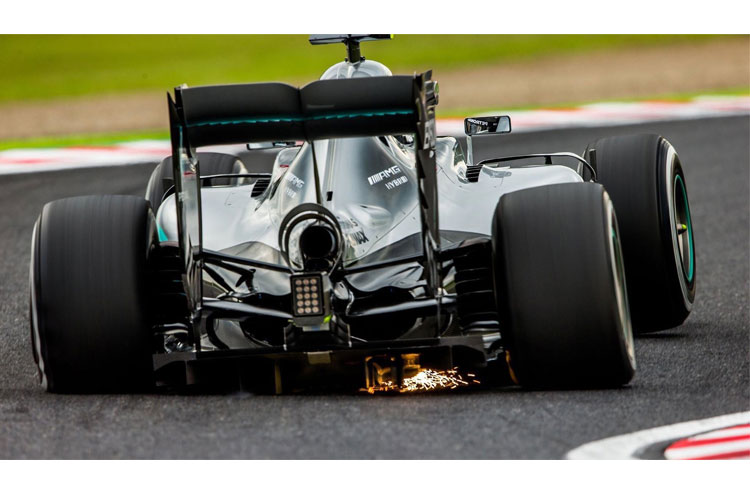With the wheel rates for the coil springs and the anti-roll bars calculated we can now look at calculating our chassis roll stiffness. When a car corners, the sprung mass rotates about a roll axis between the axles and rolls. Therefore, the body of the car rolls at an angle whilst both wheels are in contact with the ground causing a change in ride heights (in theory). Therefore, we know that for a given vertical displacement of the wheel, it will experience a certain amount of spring force. However, what we do not know yet is for a given amount of body roll, how much spring force will be generated.

Therefore, we need to add in the track width of the car along with a pre-determined amount of roll to calculate the roll stiffness of the car.

The diagram below shows a car experiencing roll at an angle (A) with a track width of (t).The vertical wheel displacement for a given amount of body roll is a direct function of the track width of the car. The equation below uses trigonometry to define the relationship between the track width and the vertical wheel displacement.Where:

• Φ=Vehicle Roll Angle
• T = Track Width

Therefore for 1 degree of roll in a car with a track width of 1.4m: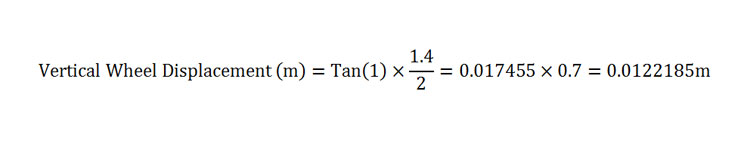For a sanity check, multiply the answer by 1000 to see it in millimetres which in this case is 12.21mm which sounds about right.

When the car is in roll the anti-roll bar and coil spring work together to produce a combined stiffness. As they are operating in parallel we can use the parallel spring equation to combine the two wheel rates to make them one wheel rate in roll using the below equation.Therefore using our above example figures for wheel rates this becomes: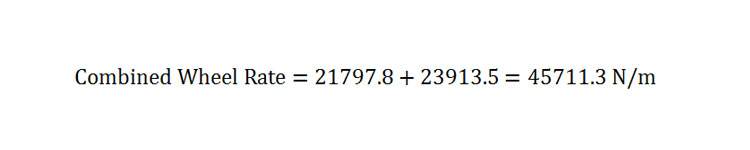With this value for a combined wheel rate in roll we can use the track width of the car as well and input the values into the below equation to generate the chassis roll stiffness per degree of roll.  This is done with the following equation:So:So far we have only been observing a single axle. We can now calculate the front chassis roll stiffness and the rear chassis roll stiffness and then the total chassis roll stiffness for this car. The figures used above were from the front springs and anti-roll bar so we effectively calculated the front chassis roll stiffness. To calculate the rear chassis roll stiffness input the rear values into the above equations. Numbers you might need to alter are:

• Track width – This is due to some cars having different front and rear track widths
• Coilover Wheel Rate – This should have been calculated in the first section for the rear as well so just input this value for the rear coil wheel rate.
• Anti-Roll Bar Wheel Rate – Again this should have been calculated in the first section so input the value for the rear anti-roll bar wheel rate.

For the purposes of this example we have a rear coil spring wheel rate of 28125 N/m and a rear anti-roll bar wheel rate of 25326 N/m. Our track width is remaining the same for the rear. This gives the below equation for the rear chassis roll stiffness:Now we can add these two roll stiffness’s together as they are working in parallel to generate the overall chassis roll stiffness for this car.Chassis StiffnessNow that we have calculated chassis roll stiffness we need to look at chassis stiffness. All of the calculations above are based upon the assumption that the chassis is rigid and has minimal flex. Chassis stiffness is the resistance of the chassis to bending or twisting during dynamic conditions. One way to consider chassis stiffness is to observe it as a single large spring; a spring that connects the front and rear suspension systems and the left and right suspension systems. Adding this spring into the equation introduces multiple more complex equations to begin trying to close in on spring rates for your car.

It is possible to measure the chassis stiffness of your car with the correct equipment. The image above shows a professional set up to calculate chassis stiffness but is very expensive to use and far more expensive to buy. The equipment required for a DIY set up is:

• 2 Scales
• 2 Jack Screws
• Chassis Frame
• Wheel Stands (Knife Edges)
• Dial Indicators (around 6)
• Support Chains

The diagram below from Milliken and Milliken shows how all of these parts can be assembled on the car to form a chassis stiffness testing rig.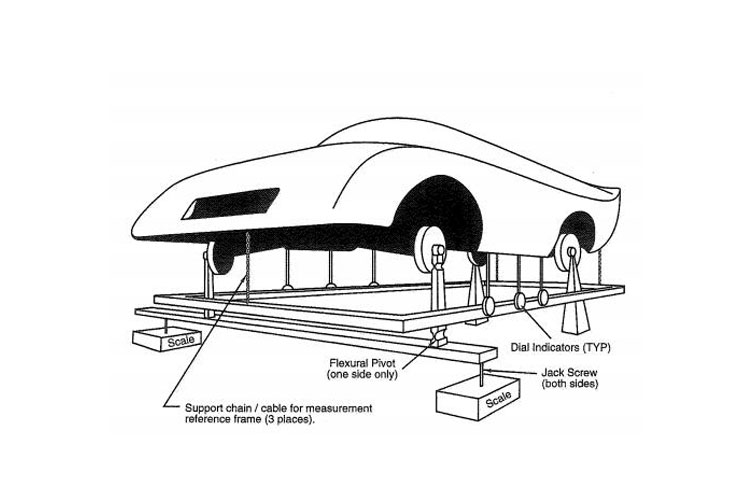All dampers must be blocked out by solid extremely stiff bar replacements. The frame needs to be suspended from the car at a height where the dial indicators are in the centre of their range and in contact with the sills of the car at equal heights.

If your vehicle has leaf springs at the rear then these need replacing with a large very stiff bar that attaches in the leaf spring mounting points.

From each wheel the knife edge stands come down. The rear wheels must be firmly supported so they are unable to lift. The front wheels are mounted on knife edge stands which are then mounted on a stiff horizontal beam. This beam is suspended from the scales by means of 2 screw jacks.

Torque can now be applied to the chassis by screwing one of the screw jacks upwards. The scale reading should be noted down before hand when at zero torque. For each adjustment, note down the scale readings along with the dial indicator readings at that stage. Do this until a maximum torque is found.

To calculate the torque applied, half the difference between the scale readings (in kg) and multiply it by the distance between the front knife edges (in meters). Finally multiply this number by the constant 9.81 to get your torque applied in newtons per meter.

Many chassis stiffness tests result in a relatively linear curve when the results are plotted on a graph of torque vs degrees of twist. Therefore we can use the results to get a figure for our chassis stiffness.

Many dial gauges measure in inches so for this equation we will assume that is the case. If it is not then use the conversion of 1 inch=25.4mm. To convert the dial gauge readings from inches into degrees, the proportionality of every 1inch in 57.3inches is equal to 1 degree can be used. Therefore if the dial indicators show a difference of 0.05 inches and the pair of dial indicators in question are 1.2m apart or 47.25 inches then the amount of twist is equal to:We also know the torque that was applied to the chassis to generate this amount of twist. For example, if 2150Nm of torque was applied to generate 0.06 degrees of twist. We can use the below equation to generate value for the amount of torque required to twist our chassis by 1 degree as this is the industry standard units for chassis stiffness.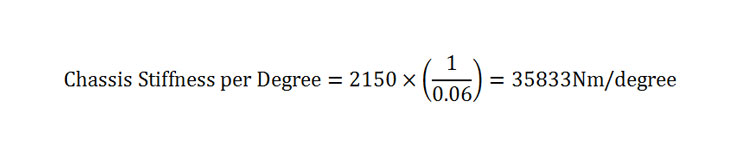Effects of Chassis Stiffness on Roll StiffnessThe chassis roll stiffness equations using the anti-roll bars and the coil rates were calculated assuming that the chassis has no flexibility. To include the chassis flex would take a lot more equations and a much more complex process. However, how much does the chassis stiffness actually affect the overall roll stiffness of a car? And is there a certain minimum stiffness that we can meet to be able to accurately remove the chassis stiffness from the equations?

A study was carried out by four American university lecturers that was approved/published by SAE International which investigated “The Effects of Chassis Flexibility on Roll Stiffness”. After carrying out multiple physical and computational tests on different chassis and suspension arrangements they were able to conclude that there is a minimum chassis stiffness that can be reached in order to accurately remove chassis stiffness from equations relating to roll stiffness.

Their conclusion was that “the minimum torsional stiffness required so that the effective roll stiffness of the front suspension is within 3% from the roll stiffness with a rigid chassis, is 31320 Nm/degree. This level of chassis torsional stiffness is sufficient to ensure that roll stiffness between sprung and un-sprung masses is due almost entirely to the suspension.”

Looking at our calculation for a chassis above it is stiffer than the minimum requirement and we can therefore rule it out. If you have measured your chassis stiffness and it is below this value then it is best to install further bracing to your car in the form of aftermarket bolt on braces or the installation of a roll cage etc.

### 12 thoughts on “Wheel Rate and Chassis Roll Stiffness”

1.Martin says:

Hi ! I am a motorsport student. How have you calculated the anti-roll bar spring rate in N/m which is used for calculating the ARB wheel rate?

Best Regards !

2.Solihin says:

Hi, I am Mechanical Eng student.Just wanna ask how to calculate the wheel rate for solid axle suspension system?

1.Suspension Secrets says:

Hi, thanks for the comment. The manual route would be best for you to measure the wheel displacement and spring displacement at the same time whilst working the wheel through full travel. You can then calculate wheel rate with the known spring rate and the installation ratio.

Thanks

3.sayyed jamal says:

Hi, thanks for your perfect site 🙂

I’m PhD student in mechanical engineering in dynamic, vibration and control field.

I found a mathematical method based on virtual work principles for the wheel-rate and wheel-shock exact analytical calculations.

I want to experimentally verify my method but I don’t have any equipment such as sensors, actuators and etc.

Can your institute help me to do this verification?
There is a big gap in this subject, so we will be able to publish a valuable paper.

1.Suspension Secrets says:

Hi Sayyed, thanks for the comment. That sounds very interesting. Unfortunately this is not something we would be able to help with but is something that your university can hopefully provide the resources for.

Thanks

4.John says:

Very good article.Thanks.But how could i apply these to a Formula Vee racing car.Using VW Beetle front suspension and Zero Roll at rear with single radius rods going to rear of chassic.

1.Suspension Secrets says:

Hi John, thanks for the comment. With a fixed rear beam axle the roll centre is located in the centre of the axle. From there the equations should work the same for you.

Thanks

5.Tim says:

Hi, thankyou for this post, it contains some great information, is it possible to calculate the effect of changing the roll centre height on roll stiffness? From the equations above it seems like the only variable that will be affected is the change in the motion ratio (if for example we change rear pickup points on a gearbox for double wishbone suspension).

My understanding of changing the roll centre height means that we are changing the moment arm for weight to be transferred under lateral acceleration, so if we increase the roll centre height that effectively means there is a greater moment arm for the weight to be transferred through which would result in a lower roll stiffness

I read the other article that was posted about roll centre and roll moment, but this would suggest the only way to calculate it would be to know the chassis roll angle at a particular lateral acceleration, then calculate the chassis stiffness by calculating the torque (with the variable being the change in distance between to CoG and the roll centre), but obtaining the roll angle seems like quite a difficult task

Thank you!

1.Suspension Secrets says:

Hi Tim, thanks for the comment. Obtaining roll angle is a task that requires quite a lot of measurement equipment. However, this is the best route for accurately calculating roll moment. The other route would be to draw out your roll centres with a scale drawing or on CAD and then use G data from a data logger to apply to the COG and therefore calculate roll moment using this data.

Thanks

6.Gary Lapidus says:

Hi,
Your first equation for Wheel Rate contains “Installation Ratio”; is this a variable or is it a constant? You assign a value of 0.6 to Installation Ratio, but assuming it’s a variable there’s no explanation shown. Can you please expand on this?
Thanks.

1.Suspension Secrets says:

HI Gary,

The installation ratio is a calculated ratio of the installation of your suspension. It is specific to your car. Please see our article covering installation ratio and how to calculate it.

Many Thanks